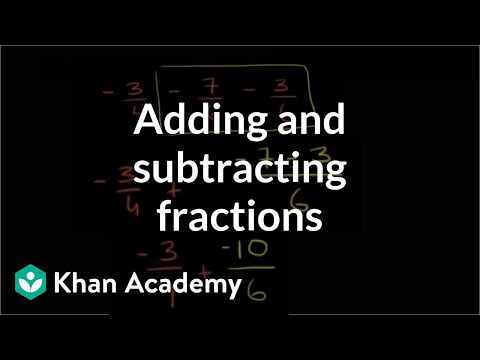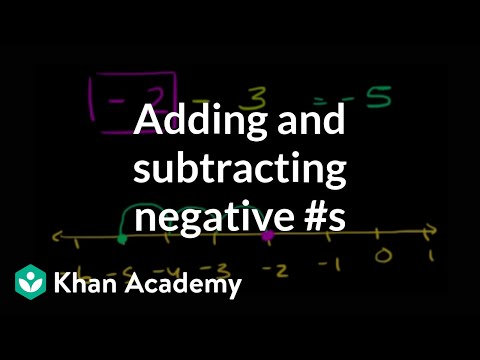Video

# Adding negative numbers on the number line (Full video)

Khan Academy

## Supporting grades: 7

Description: Sal shows how to model 6 + (-2) using vertical number lines. So we've already spent some time introducing ourselves to the idea of adding or subtracting positive and negative numbers. What I now what do in this video is do a bunch of examples using the adding negative numbers on the number line exercise on Khan Academy, so that we can think about different ways to model, or think about, or visualize adding and subtracting positive and negative numbers. This number line model I guess we could call it, it's saying this is positive two right over here.

You must log inorsign upif you want to:*

*Teacher Advisor is 100% free.

### Other videos you might be interested in### Adding & subtracting fractions (Full video)

#### Khan Academy